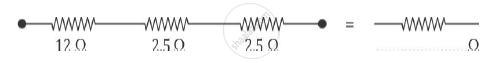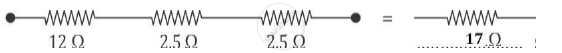# Complete the Following - Science and Technology 1

Complete the following :-

(a)#### Solution

(a)The equivalent resistance is Rs = 12Ω + 2.5Ω + 2.5Ω = 17Ω

Concept: System of Resistors - Resistors in Series
Is there an error in this question or solution?